# GCSE Maths Foundation Papers

Here you will find all our GCSE maths foundation papers, created in the style of GCSE maths foundation past papers from Edexcel, AQA and OCR.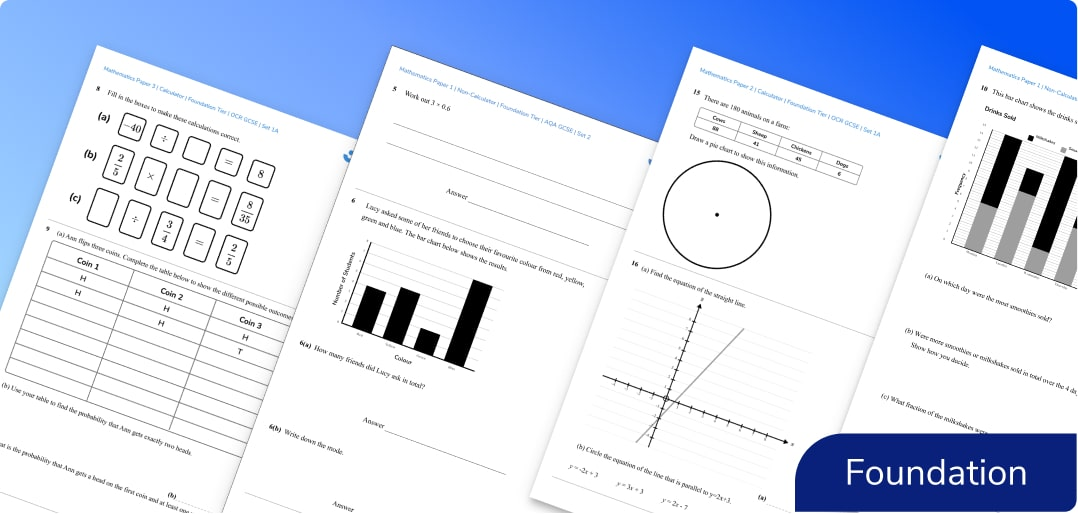What can you expect from the GCSE maths foundation papers?

Each set contains three GCSE exam papers: Foundation Paper 1 (non-calculator), Foundation Paper 2 (calculator), Foundation Paper 3 (calculator).

Exam questions are set out in the style of the specimen papers from the exam boards and include answers, mark schemes and grade boundaries.

Sets 1a and 1b are based on the foundation level GCSE mathematics advanced information 2022.

Sets 2 onwards (more to come) are predicted papers based on GCSE maths past papers and the foundation tier specification for each board.

## How to use these GCSE maths foundation papers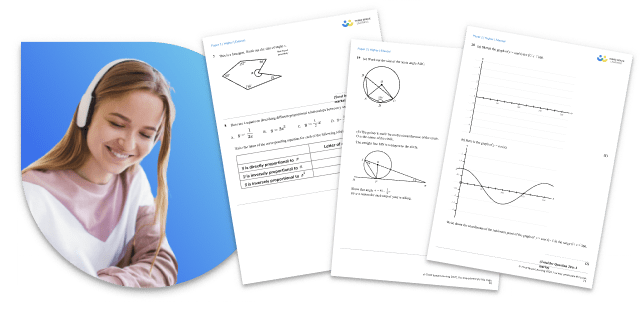These specially created GCSE maths foundation papers are an essential addition to your GCSE maths revision for the foundation tier maths course of most exam boards including WJEC and IGCSE maths.

They closely match the past GCSE maths papers provided by the AQA, Edexcel & OCR exam boards. All maths questions on the exam papers are created by current or former AQA, Edexcel and OCR examiners and expert maths teachers.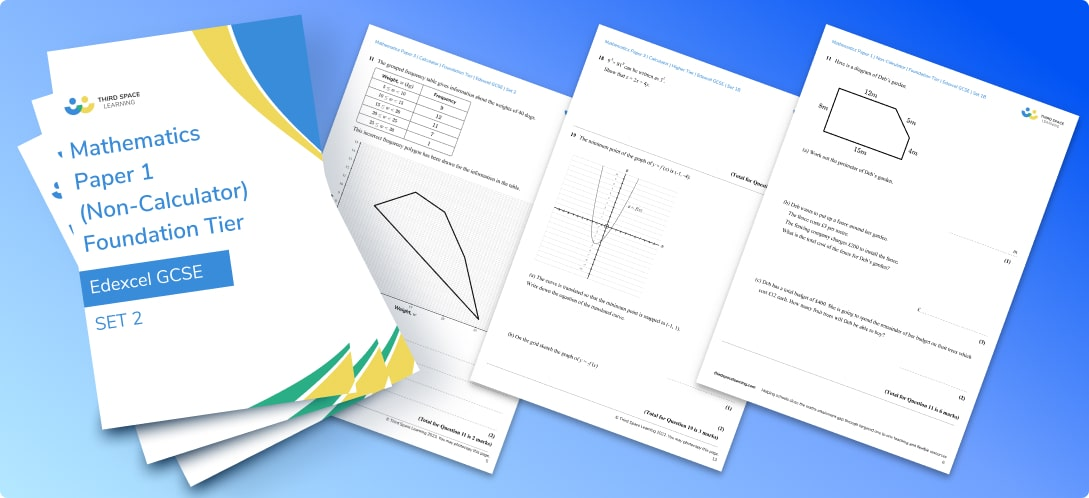While the style and layout of these question papers will be familiar to students the content is new and unique.

Schools often use these GCSE maths foundation papers as maths practice papers and mock exams as the mark scheme and coverage (including topics like area and perimeter, angles in polygons, and decimals fractions and percentages) enable you also to see individual gaps for each student.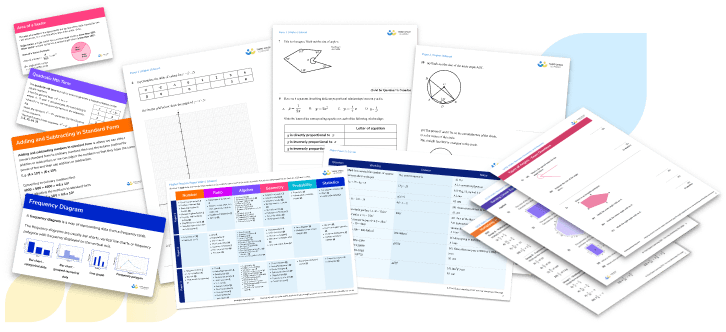If you need further GCSE level maths exam practice and preparation, take a look at the free worksheets and revision resources available below. As one to one online maths tutor specialists, we know how to support students in preparation for GCSEs.

Looking for higher tier maths? We also provide free GCSE Maths Higher Papers for those students aiming for grades 5-9.

More GCSE Past Papers

GCSE Maths Papers & Past Papers

OCR Maths Papers & Past Papers

Edexcel Maths Papers & Past Papers

AQA Maths Papers & Past Papers

For old spec past exam papers and the AQA GCSE maths, OCR GCSE maths and Edexcel GCSE maths past papers follow these links:

Edexcel

AQA

OCR

## Can't find what you're looking for?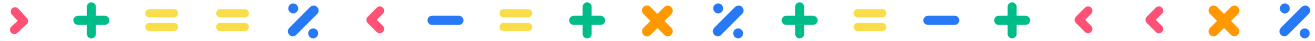## Teaching support from the UK’s largest provider of in-school maths tuition

At Third Space Learning, we’re on a mission to help teachers like you shape your students into confident, able mathematicians.

Request a quote